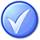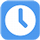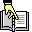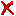Please review your test results by moving cursor to answer sheet icon.1.A)
B)
C)
D)

Your answer=, Correct answer=,

2.A)
B)
C)
D)

Your answer=, Correct answer=,

3.A)
B)
C)
D)

Your answer=, Correct answer=,

4.You are beginning a new project. When should you use the Perform Integrated Change Control process?

A) Throughout the entire project
B) Only when closing out the project
C) Only after the project is completely funded
D) Only after the project scope is clearly definedYour answer=C, Correct answer=, Work Sheet:

5.A)
B)
C)
D)

Your answer=, Correct answer=,